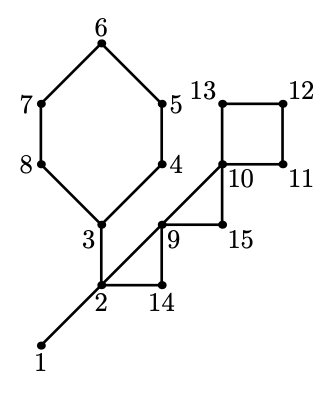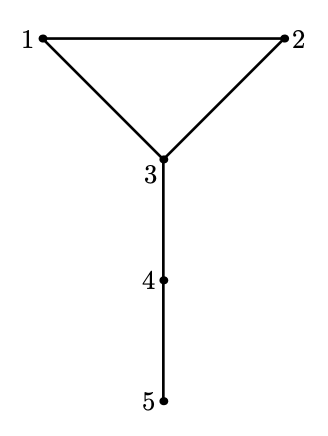시간 제한 메모리 제한 제출 정답 맞은 사람 정답 비율
4 초 512 MB 23 13 11 61.111%

## 문제

If you want to make an array problem harder — solve it on a tree. If you want to make a tree problem harder — solve it on a cactus

Conventional wisdom

NEERC featured a number of problems in previous years about cactuses — connected undirected graphs in which every edge belongs to at most one simple cycle. Intuitively, a cactus is a generalization of a tree where some cycles are allowed. An example of a cactus from NEERC 2007 problem is given on the picture below.You are playing a game on a cactus with Chloe. You are given a cactus. Chloe had secretly picked one vertex v from the cactus and your goal is to find it. You can make at most 10 guesses. If your guess is vertex v — you win. Otherwise, if your guess is another vertex u — Chloe helps you and tells you some vertex w which is adjacent to u and such that the distance from w to v is strictly less than the distance from u to v (here the distance is the number of edges in the shortest path between vertices).

## 프로토콜

First, the testing system writes a line with two integers n and m (1 ≤ n ≤ 500; 0 ≤ m ≤ 500). Here n is the number of vertices in the graph. Vertices are numbered from 1 to n. Edges of the graph are represented by a set of edge-distinct paths, where m is the number of such paths. Each of the following m lines contains a path in the graph. A path starts with an integer ki (2 ≤ ki ≤ 500) followed by ki integers from 1 to n. These ki integers represent vertices of a path. Adjacent vertices in a path are distinct. The path can go to the same vertex multiple times, but every edge is traversed exactly once in the whole input. The graph in the input is a cactus.

To prove that your program can find a secret vertex in at most 10 queries, you need to do that n times. Each time testing system picks some vertex before interacting with your program. Your program makes guesses by writing lines with a single number u (1 ≤ u ≤ n).

Testing system responds by writing lines with one of the two responses:

• “FOUND” — means that your guess is correct. After that, your program should proceed to the next test case or terminate if n vertices were already guessed.
• “GO w” — means that your guess is incorrect, but now you know that the distance from vertex w to the secret vertex is less than the distance from u. It is guaranteed that vertices u and w are connected by an edge.

Do not forget to flush the output after each guess!

## 예제 입력 1

5 2
5 1 2 3 4 5
2 1 3

FOUND

GO 4

FOUND

GO 2

FOUND

GO 1

FOUND

GO 4

GO 5

FOUND


## 예제 출력 1



3

3

4

3

2

3

1

3

4

5## 힌트

Empty lines are added to the standard input and the standard output examples for clarity only. They are not present during the actual interaction.

## 채점 및 기타 정보

• 예제는 채점하지 않는다.